# Black-Scholes Excel Formulas and How to Create a Simple Option Pricing Spreadsheet

This page is a guide to creating your own option pricing Excel spreadsheet, in line with the Black-Scholes model (extended for dividends by Merton). Here you can get a ready-made Black-Scholes Excel calculator with charts and additional features such as parameter calculations and simulations.

## Black-Scholes in Excel: The Big Picture

If you are not familiar with the Black-Scholes model, its assumptions, parameters, and (at least the logic of) the formulas, you may want to read those pages first (overview of all Black-Scholes resources is here).

Below I will show you how to apply the Black-Scholes formulas in Excel and how to put them all together in a simple option pricing spreadsheet. There are four steps:

1. Design cells where you will enter parameters.
2. Calculate d1 and d2.
3. Calculate call and put option prices.
4. Calculate option Greeks.

## Black-Scholes Inputs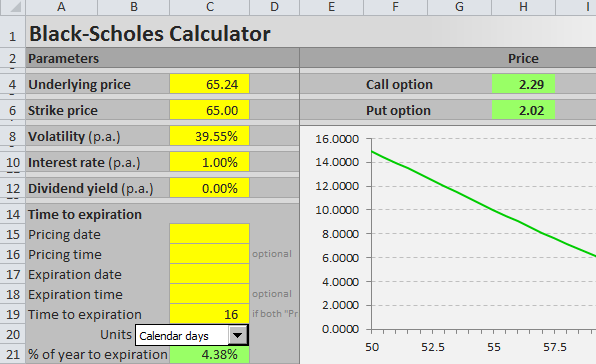First you need to design six cells for the six Black-Scholes parameters. When pricing a particular option, you will have to enter all the parameters in these cells in the correct format. The parameters and formats are:

S = underlying price (USD per share)
K = strike price (USD per share)
σ = volatility (% p.a.)
r = continuously compounded risk-free interest rate (% p.a.)
q = continuously compounded dividend yield (% p.a.)
t = time to expiration (% of year)

Underlying price is the price at which the underlying security is trading on the market at the moment you are doing the option pricing. Enter it in dollars (or euros/yen/pound etc.) per share.

Strike price, also called exercise price, is the price at which you will buy (if call) or sell (if put) the underlying security if you choose to exercise the option. If you need more explanation, see: Strike vs. Market Price vs. Underlying Price. Enter it also in dollars per share (it must have same units as underlying price, also with the same contract or lot multipliers).

Volatility is the most difficult parameter to estimate (all the other parameters are more or less given). It is your job to decide how high volatility you expect and what number to enter – neither the Black-Scholes model, nor this page will tell you how high volatility to expect with your particular option (for more on that, see the volatility tutorials, particularly historical and implied volatility). Being able to estimate (= predict) volatility with more success than other people is the hard part and key factor determining success or failure in option trading. The important thing here is to enter it in the correct format, which is % p.a. (percent annualized).

Risk-free interest rate should be entered in % p.a., continuously compounded. The interest rate's tenor (time to maturity) should match the time to expiration of the option you are pricing. You can interpolate the yield curve to get the interest rate for your exact time to expiration. Interest rate does not affect the resulting option price very much in the low interest environment that we've had in the recent years, but it can become very important when rates are higher (for more details on the effect of interest rates on option prices see the option rho tutorial).

Dividend yield should also be entered in % p.a., continuously compounded. If the underlying stock doesn't pay any dividend, enter zero. If you are pricing an option on securities other than stocks, you may enter the second country interest rate (for FX options) or convenience yield (for commodities) here.

Time to expiration should be entered as % of year between the moment of pricing (now) and expiration of the option. For example, if the option expires in 24 calendar days, enter 24/365 = 6.58%. Alternatively, you can measure time in trading days rather than calendar days. If the option expires in 18 trading days and there are 252 trading days per year, you will enter time to expiration as 18/252 = 7.14%. You can also be more precise and measure time to expiration to hours or even minutes. In any case you must always express the time to expiration as % of year in order for the calculations to return correct results (it is very easy in Excel – just divide the number of days to expiration by the number of days per year).

I will illustrate the calculations on the example below. The parameters are in cells A44 (underlying price), B44 (strike price), C44 (volatility), D44 (interest rate), E44 (dividend yield), and G44 (time to expiration as % of year).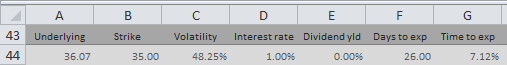Note: It is row 44, because I am using the Black-Scholes Calculator for screenshots and it has charts in the rows above. You can of course start in row 1 or arrange your calculations in a column.

## Black-Scholes d1 and d2

When you have the cells with parameters ready, the next step is to calculate d1 and d2, because these terms then enter all the calculations of call and put option prices and Greeks. The formulas for d1 and d2 are: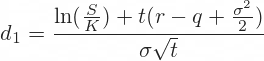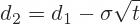All the operations in these formulas are relatively simple mathematics. The only things that may be unfamiliar to some less savvy Excel users are the natural logarithm (`LN` Excel function) and square root (`SQRT` Excel function).

The hardest thing with the d1 formula is making sure you put the brackets in the right places. This is why you may want to calculate individual parts of the formula in separate cells, as I do in the example below: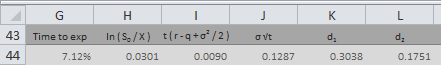First I calculate the natural logarithm of the ratio of underlying price and strike price (this is why they must have the same units) in cell H44:

``=LN(A44/B44)``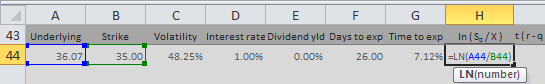Then I calculate the rest of the numerator of the d1 formula in cell I44:

``=(D44-E44+POWER(C44,2)/2)*G44``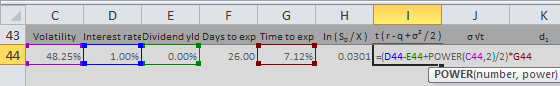Then I calculate the denominator of the d1 formula in cell J44. Another reason why you may want to calculate d1 in separate parts is that this term will also enter the formula for d2:

``=C44*SQRT(G44)``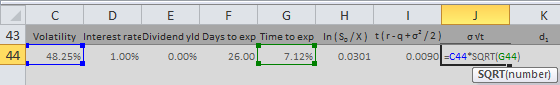Now I have all the three parts of the d1 formula and I can combine them in cell K44 to get d1:

``=(H44+I44)/J44``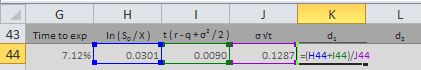Finally, I calculate d2 in cell L44:

``=K44-J44``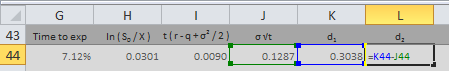## Black-Scholes Option Price Excel Formulas

The Black-Scholes formulas for call option (C) and put option (P) prices are: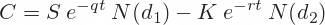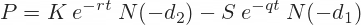The two formulas are very similar. There are four terms in each formula. I will again calculate them in separate cells first and then combine them in the final call and put formulas.

### N(d1), N(d2), N(-d2), N(-d1)

Potentially unfamiliar parts of the formulas are the N(d1), N(d2), N(-d2), and N(-d1) terms.

N(x) denotes the standard normal cumulative distribution function: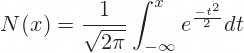For example, N(d1) is the standard normal cumulative distribution function for the d1 that we have calculated in the previous step.

In Excel you can easily calculate the standard normal cumulative distribution functions using the `NORM.DIST` function, which has four parameters:

``NORM.DIST(x, mean, standard_dev, cumulative)``
• x = link to the cell where you have calculated d1 or d2 (with minus sign for -d1 and -d2)
• mean = enter 0, because it is standard normal distribution
• standard_dev = enter 1, because it is standard normal distribution
• cumulative = enter TRUE, because it is cumulative

For example, I calculate N(d1) in cell M44:

``=NORM.DIST(K44,0,1,TRUE)``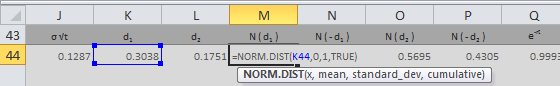Note: There is also the `NORM.S.DIST` function in Excel, which is the same as `NORM.DIST` with fixed mean = 0 and standard_dev = 1 (therefore you enter only two parameters: x and cumulative). You can use either. `NORM.S.DIST` may not be available in some spreadsheet software.

### The Terms with Exponential Functions

The exponents (e-qt and e-rt terms) are calculated using the `EXP` Excel function with `-q*t` or `-r*t` as parameter.

I calculate e-rt in cell Q44:

``=EXP(-D44*G44)``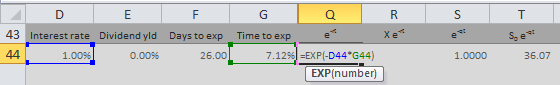Then I use it to calculate K e-rt in cell R44:

``=B44*Q44``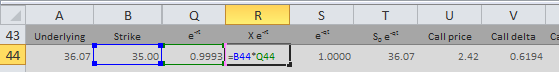Analogically, I calculate e-qt in cell S44:

``=EXP(-E44*G44)``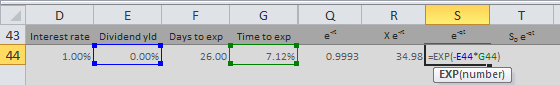Then I use it to calculate S e-qt in cell T44:

``=A44*S44``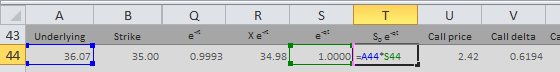Now I have all the individual terms and I can calculate the final call and put option price.

### Call Option PriceI combine the four terms in the call formula to get call option price in cell U44:

``=T44*M44-R44*O44``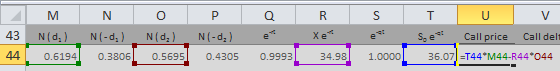### Put Option PriceI combine the four terms in the put formula to get put option price in cell U44:

``=R44*P44-T44*N44``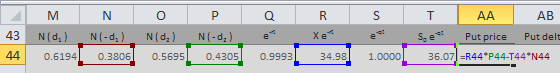## Black-Scholes Greeks in Excel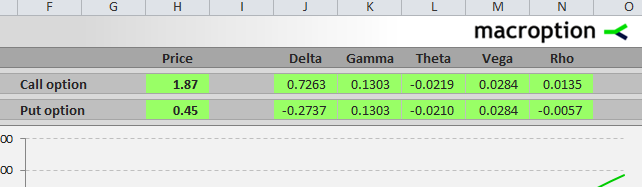Here you can continue to the second part of this tutorial, which explains Excel calculation of the Greeks: delta, gamma, theta, vega, and rho:

Continue to Option Greeks Excel Formulas

Or you can see how all the Excel calculations work together in the Black-Scholes Calculator. Explanation of the calculator's other features (parameter calculations and simulations of option prices and Greeks) are available in the calculator's user guide.

By remaining on this website or using its content, you confirm that you have read and agree with the Terms of Use Agreement.

We are not liable for any damages resulting from using this website. Any information may be inaccurate or incomplete. See full Limitation of Liability.

Content may include affiliate links, which means we may earn commission if you buy on the linked website. See full Affiliate and Referral Disclosure.

We use cookies and similar technology to improve user experience and analyze traffic. See full Cookie Policy.